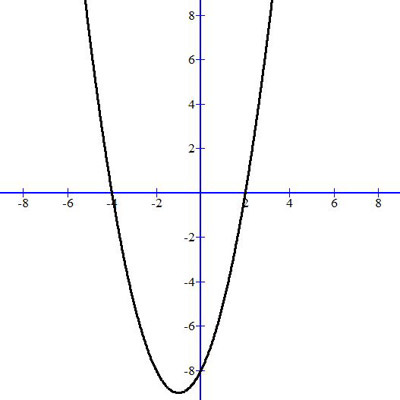##### Print Instructions

NOTE: Only your test content will print.
To preview this test, click on the File menu and select Print Preview.

See our guide on How To Change Browser Print Settings to customize headers and footers before printing.

Print Test (Only the test content will print)

1.
Which equation represents the graph of the parabola?1. $y = - x/2$
2. $y = x/2$
3. $y = -(x^2)/2$
4. $y = (x^2)/2$
2.
Graph the equation. Label the vertex and the axis of symmetry. $y= x^2 + 2$3.
Which equation represents the graph?1. $y = x$
2. $y = x^2$
3. $y = - x$
4. $y = - x^2$
4.
Which equation could describe this graph?1. $y=(x-1)(x-8)$
2. $y=(x-4)(x+2)$
3. $y=(x-2)(x+4)$
4. $y=(x+1)(x+8.5)$
5.
Graph: $y=-(x+3)^2+4$6.
Identify the axis of symmetry for the graph.1. x = 1
2. x = 0
3. y = 0
4. none of the above
7.
Graph the following equation:
$f(x) = x^2 - 3$8.
Is the discriminant positive, negative, or zero?1. Positive
2. Negative
3. Zero
9.
Graph the following equation. Label the vertex and the axis of symmetry. $y= -1/2x^2 + 2x + 3$10.
Which equation represents the graph of the parabola?1. $y = 1/2x$
2. $y =-1/2x$
3. $y = 1/2x^2$
4. $y=-1/2x^2$You need to be a HelpTeaching.com member to access free printables.